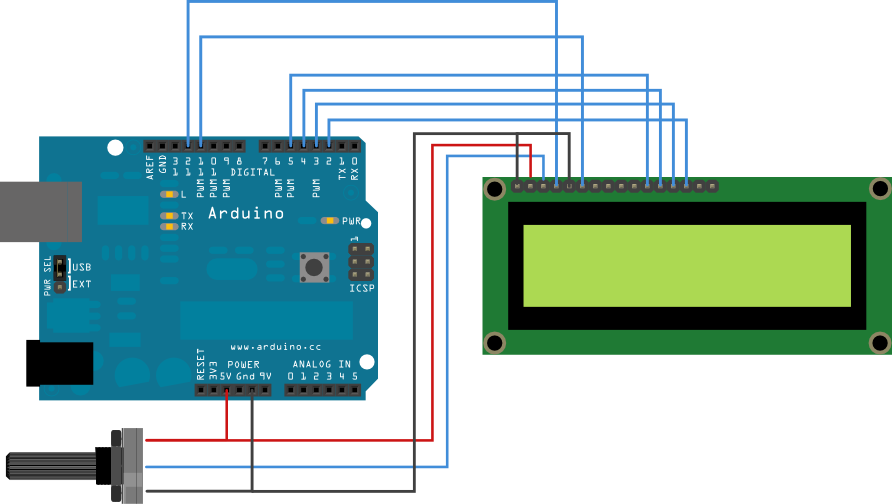Arduino

## LCD Management

It easy to see word, phrases, data, etc. on a LCD display

I used a 2x16 display.

The ciscuit diagram for connection to a generic display you can find easly online for example in the tutorial of Arduino as follow.In my case the display is a 1602B

I not know so muth about display, but comparing the connection above with a pattern of display, I don't think you will find difficult to figure out how to connect it. For example the pin D4-D5-D6-D7 in 1602B are corresponding to pins 11-12-13-14, R / W and E respectively 5-6 and so on.

Then the potentiometer adjusts the contrast of the LCD. A simple trimmer 47k or 100k is good for test operation.

There are two connections A and K (anode and cathode) that are used for display backlighting.

From online examples and with some creativity I implemented this:

 #include LiquidCrystal lcd(7, 6, 5, 4, 3, 2); //define LCD pinint led = 13,i=2;unsigned long SendTime = 0;unsigned long S=0;bool LED=true; void setup() {   lcd.begin(16, 2);  lcd.print("Hello, World!:-)");  pinMode(led, OUTPUT); //board led blink  lcd.setCursor(0, 1);  lcd.print("T: : :");} void blink_led() { //led sub program   if (millis() > SendTime + 1000) {    SendTime = millis();    if (LED==true) {      LED=false;      digitalWrite(led, HIGH);     }    else {      LED=true;      digitalWrite(led, LOW);    }  } }   void hms() { //convert numerical value time in h m s               //time sub program  unsigned long int t=millis()/1000;  unsigned short int h,m,s;  if (t >= 3600) { //hour print    h=millis()/3600000;    if (h<10) {      lcd.setCursor(3,1);      lcd.print(h);      lcd.setCursor(2,1);       lcd.print("0");    }    else {      lcd.setCursor(2,1);      lcd.print(h);    }  }  else {    h=0;    lcd.setCursor(2,1);    lcd.print("00");  }   if (t >= 60) { //minutes print    m=millis()/60000-h*60;    if (m<10) {      lcd.setCursor(6,1);      lcd.print(m);      lcd.setCursor(5,1);       lcd.print("0");    }    else {      lcd.setCursor(5,1);      lcd.print(m);    }   }  else {    m=0;    lcd.setCursor(5,1);    lcd.print("00");  }   if (t>0) { //second print    s=millis()/1000-m*60-h*3600;    if (s<10) {      lcd.setCursor(9,1);      lcd.print(s);      lcd.setCursor(8,1);       lcd.print("0");    }    else {      lcd.setCursor(8,1);      lcd.print(s);     }  }   else {    s=0;    lcd.setCursor(8,1);    lcd.print("00");  } }   void point() {  //point sub program  if (millis()> S + 249) {    S = millis();    if (i>4) {       i=1;       lcd.setCursor(10+i,1); // lcd.setCursor(17-i,1); reverse point      lcd.print(" ");     }    lcd.setCursor(11+i,1); // lcd.setCursor(16-i,1);    lcd.print(".");     lcd.setCursor(10+i,1); // lcd.setCursor(17-i,1);    lcd.print(" ");    i++;  }} void loop() { //main program   point();  //sub program call  blink_led();   hms(); }

From the program comments, you shuld understand how it works.

Now you can make changes to get familiar and  have fun with sperimentation.

Some photos of the construction:Now a short video done long ago before changing the bad connections with the best:

VIDEO